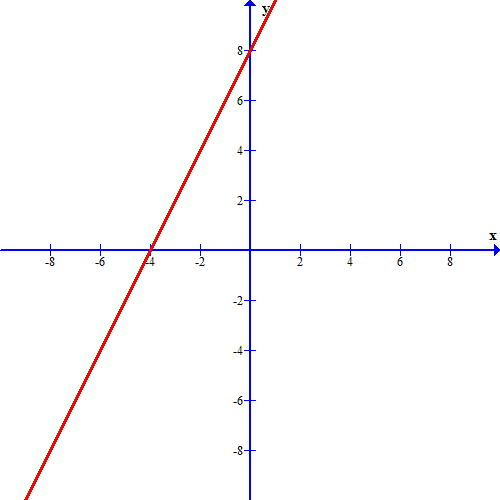# Harcourt On Core Mathematics - Algebra 1: Online Textbook Help Final Exam

Free Practice Test Instructions:

Choose your answer to the question and click 'Continue' to see how you did. Then click 'Next Question' to answer the next question. When you have completed the free practice test, click 'View Results' to see your results. Good luck!

#### Question 1 1. During the first four Summer Olympic games attended by the United States, these medal counts were awarded. Calculate the range number of medals won during these Olympic Games.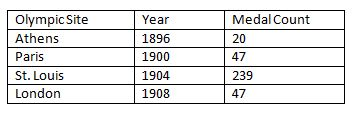#### Question 3 3. Let x = 5 and y = 4, and evaluate the expression shown using substitution.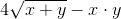#### Question 4 4. If a student got a 75, which bar should increase?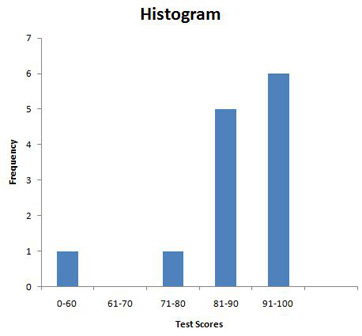#### Question 7 7. Find the domain of f(x)/g(x), if: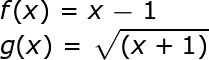#### Question 12 12. What is the equation of the graph below?